# 香港にて新たな出会い

ニコ技繋がりで香港に行く前から連絡をとり、仕事相談などをさせてもらっていた

そんな中、香港での日本人の求人を聞いたがやはりエンジニア職になると厳しい点が多い。

それを聞いて、俺はどうしようかと思ったがとりあえず実務経験1年半をどうにかして手に入れるという方針でやっていこうと思う。

それ以外にも、香港や、深センのシェアオフィスなどについても聞いてみた！

Explorium Hong Kong

サプライチェーンの未来を共に作って行こうというイノベーションハブである。

ビジネスリーダーから起業家や投資家がお互いに助け合って行こうという集まりなのだが、こんな俺が参加できるのかと思いつつ、一様コンタクトのメールを送ってみた。

また、同じ系列でデータサイエンスやAI系のイベントやプログラムもあるので引き続き調査してみます！

Bee home深圳

これはBee+系のシェアオフィスがあるみたいだ。

さらに、シェアオフィスには仮眠室がついてるので、もしかすればここで寝泊まりできるかもしれない。

ここはメーカー系の会社だ。

あまり、情報がないので回ってみるつもりだ。

これは、中国と香港の政府がお金を出し合って、青年技術者を育てるためにプロジェクトを立てたらしい。とりあえず、見に行ってみる。

テンセントとかの中国の大企業が色々お世話になっていた場所みたいだ。

# 中国に行ってみたが、中国じゃなくなっていた件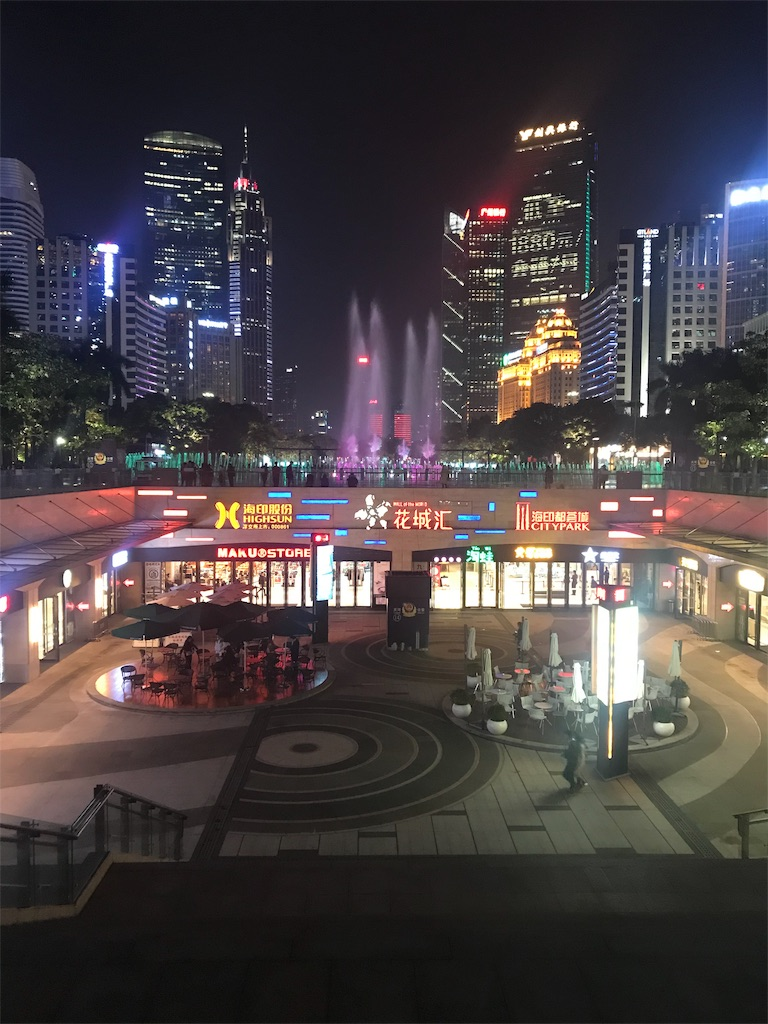広東東駅を降りてすぐ目についたのが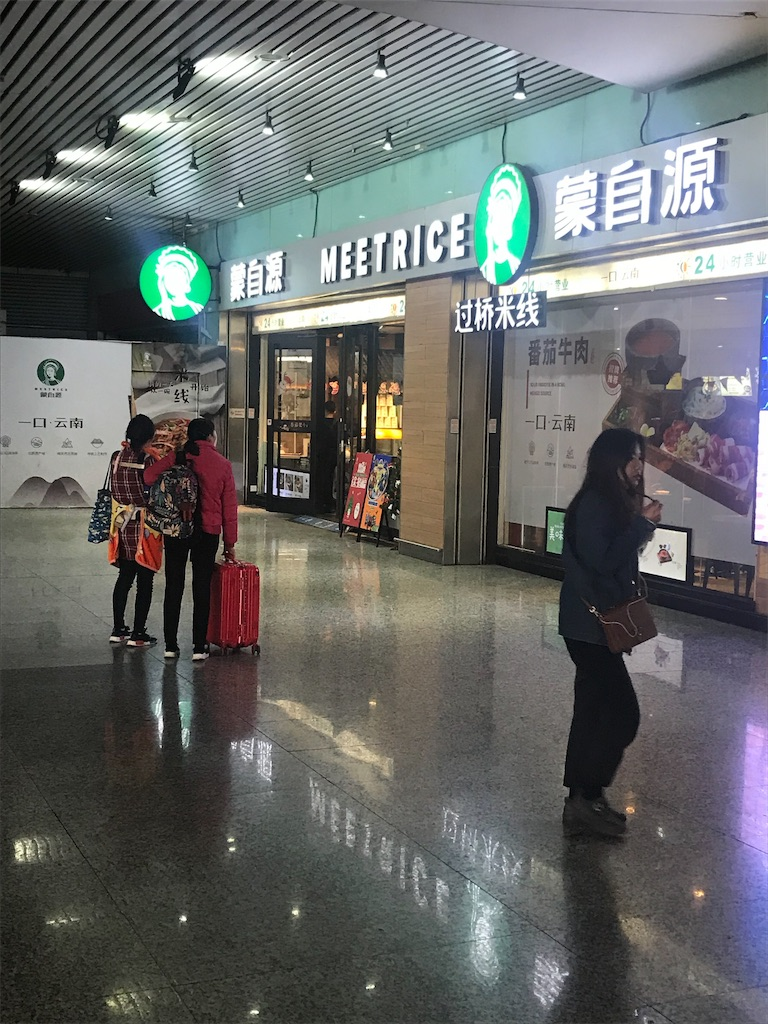スター●ックスに

そっくりの料理店「meetrice」

そして、地下鉄からホテルに向かと日本と違って、検査がある。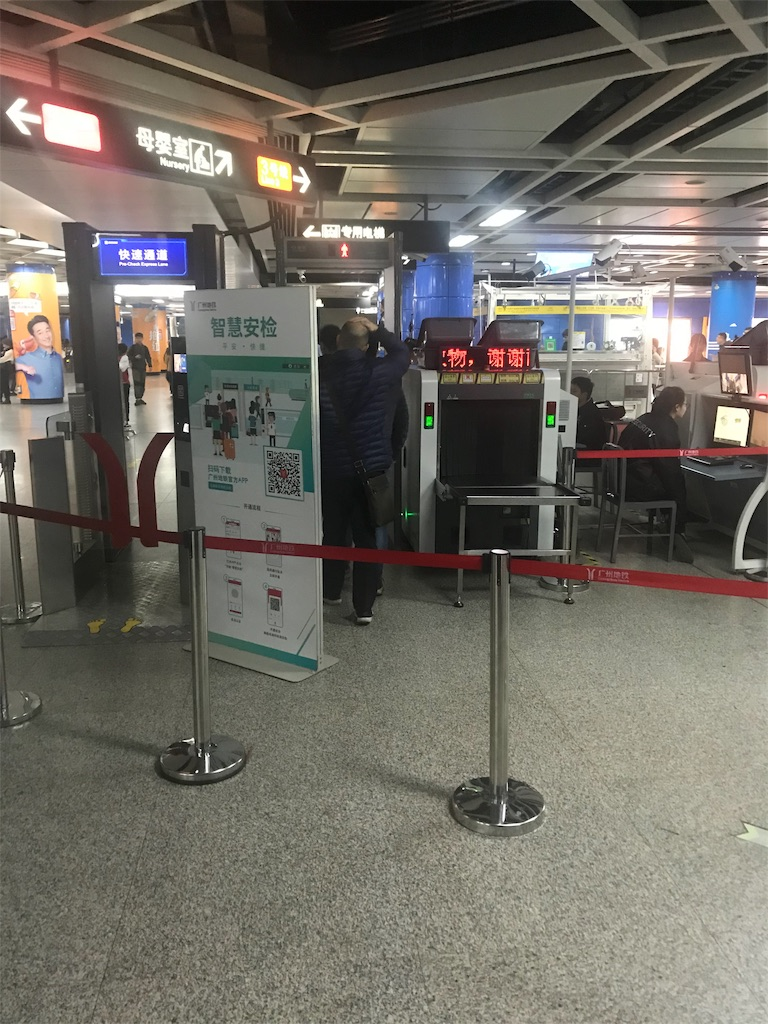カバンなどをこのゲートを通さないと

チケット売り場にすら行かしてもらえない。

まあ、基本ピーピー警報音がなっているけど全然止められない。

まあ、拳銃とかしか止めないのかと思ったけど、

そして、検査ゲートの横にたまに摩訶不思議なものがある。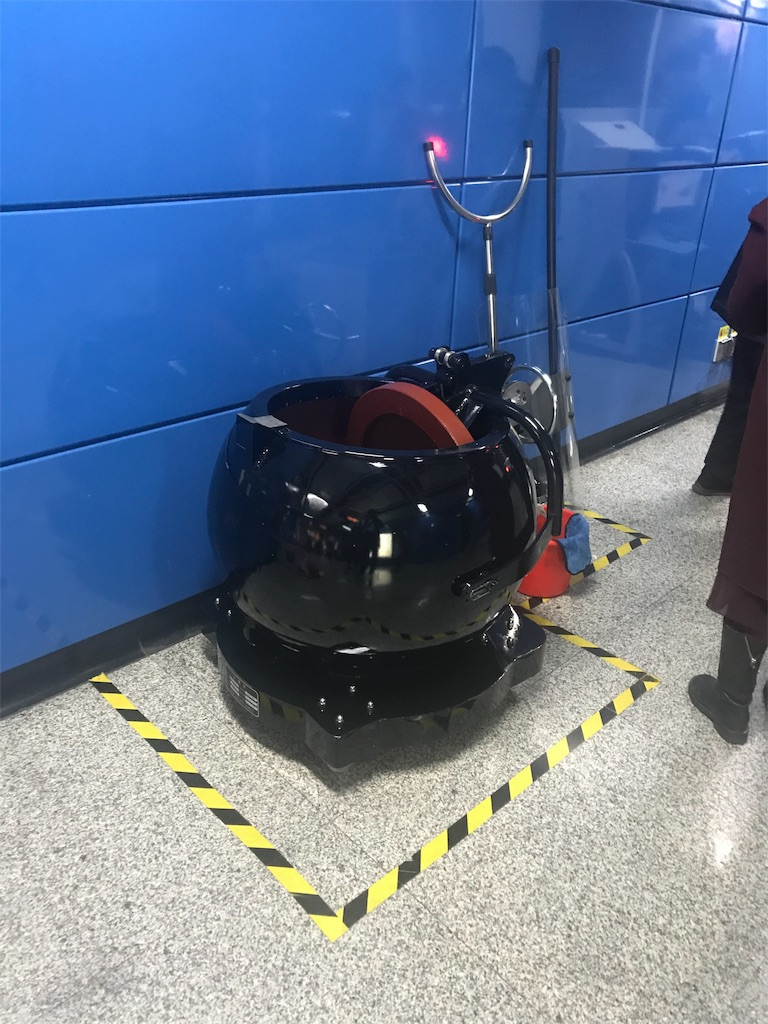そんな事あるって一瞬目を疑ったが、あるらしいたまに検査機に爆弾が検知されるからそれを即座にこの謎の箱に投げ込むらし

さすが中国、爆発するのも爆発を止めるのも一流である。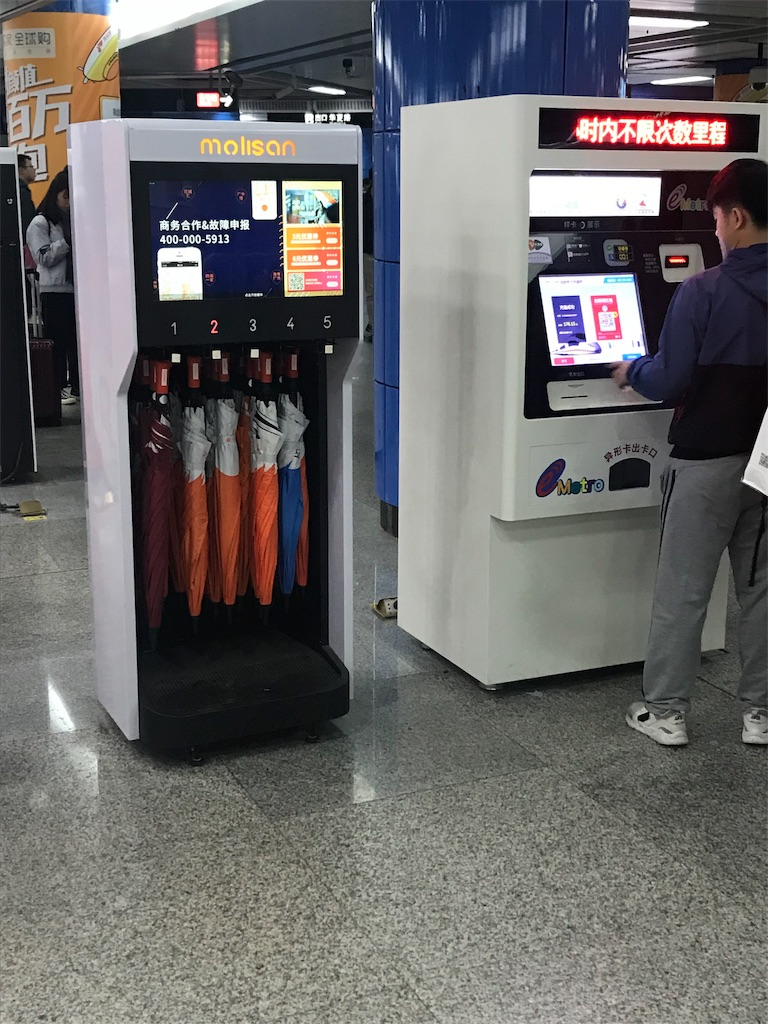また、駅に入ったらATMや自動販売機の横に傘借り機があったり、なんか中国らしいなーっと感じた。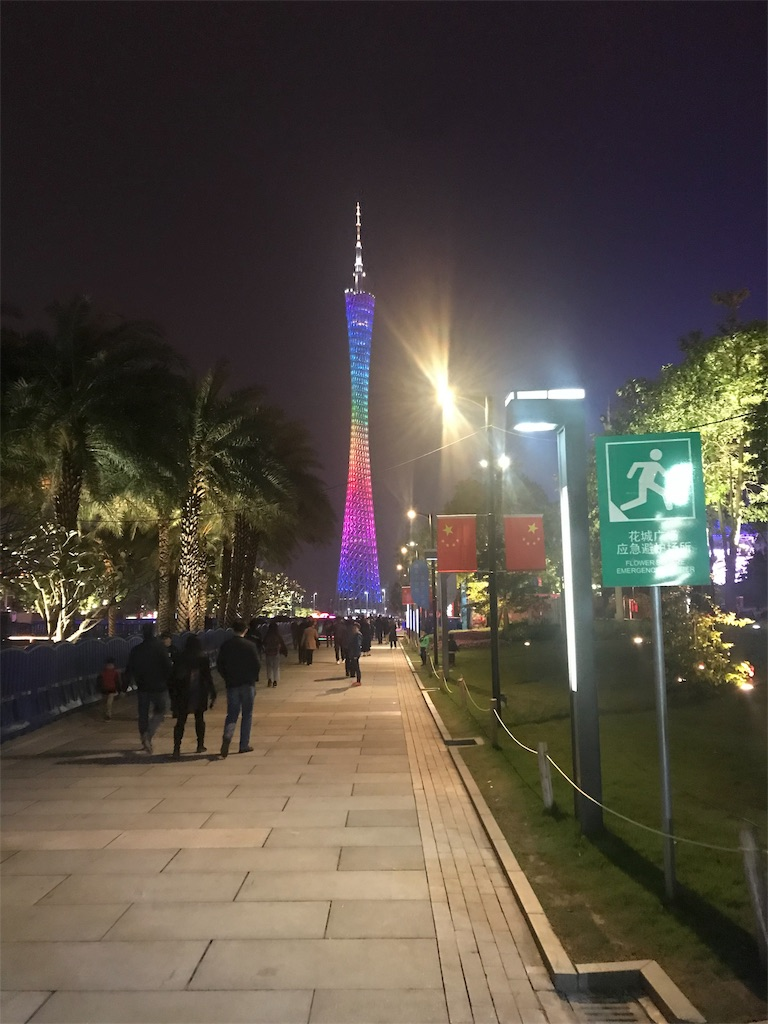ゴミひとつなく、俺が知っている中国とは全く違う別の国になっていた。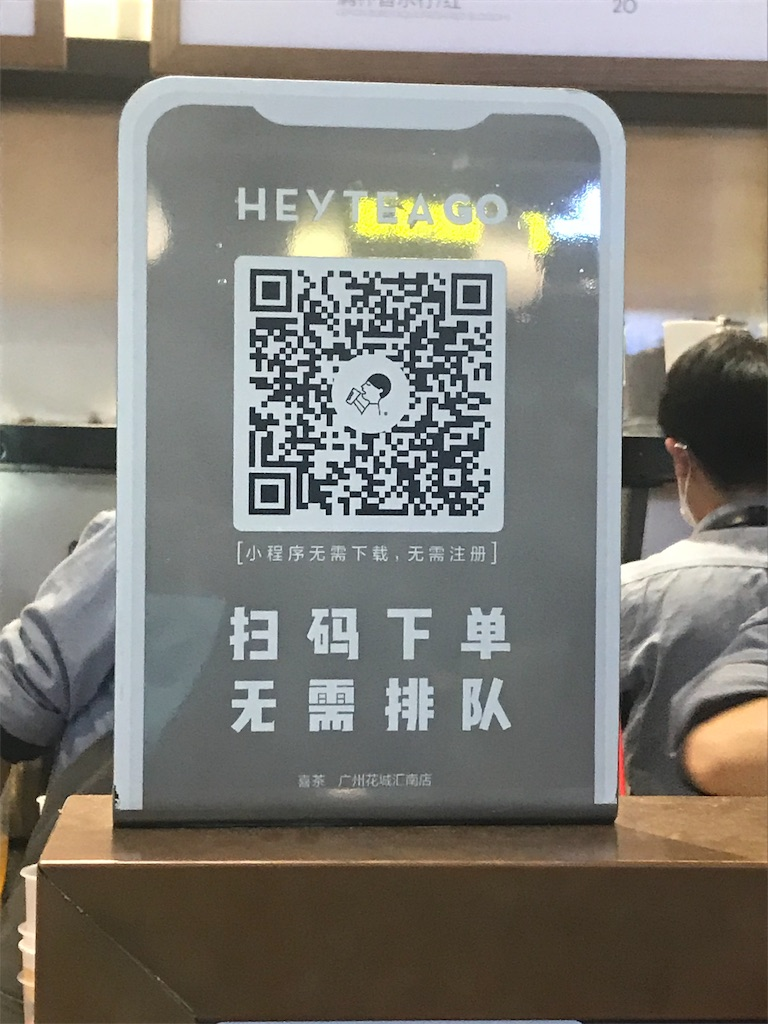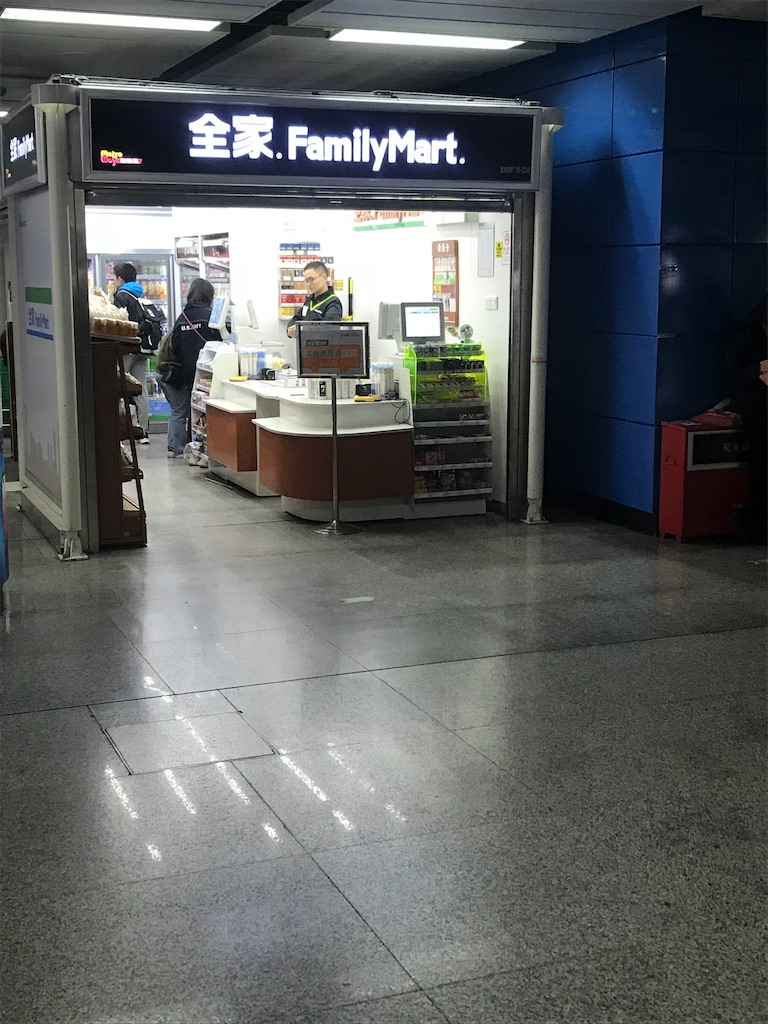タピオカミルクティーを買った時に、QRコードで出来上がったを教えてくれる。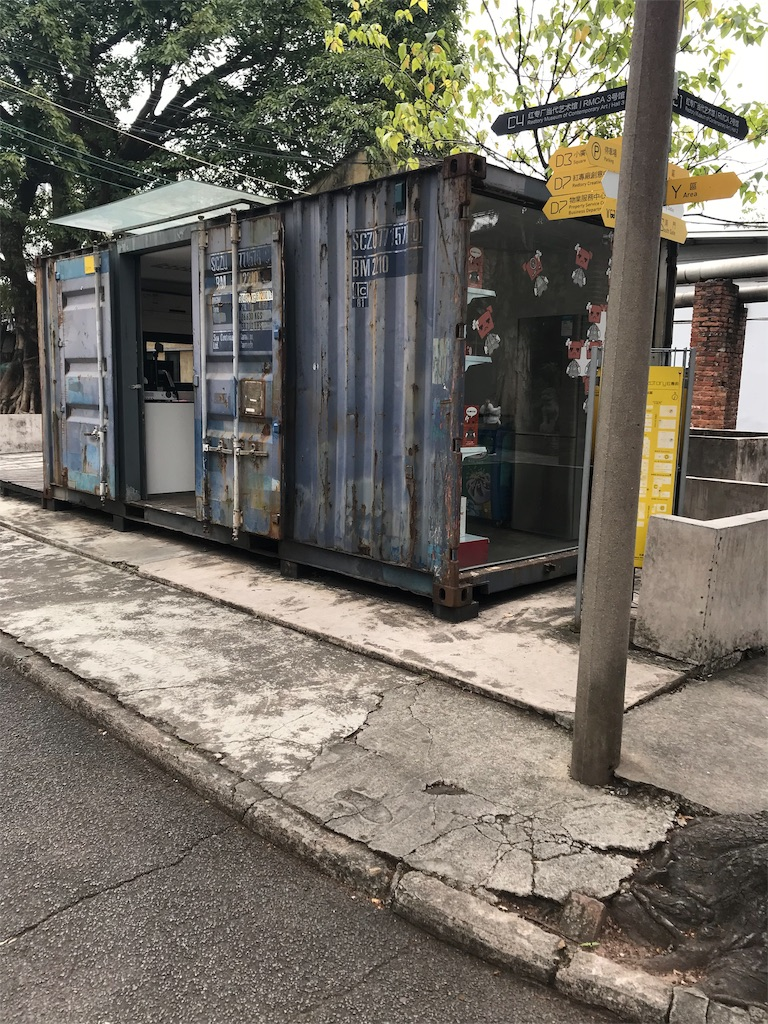かっこいい

コンテナハウスがあった。

次からは、特にIT関連の内容を発信していけたらいいなと思います

# Pythonで夏にまつわる単語の相関関係をグラフ化してみた for アドベントカレンダー

すみませんだいぶドタバタで、アドベントカレンダーを書き始めます。

ネタがないので、先週やったpythonの練習をここで披露したいと思います!!

1.まずはx,yの相関係数を求める式(find_corr_x_y)をコーディングする(practice3_5)

`def find_corr_x_y(x,y):    # if len(x) != len(y):    n = len(x)    try:        n2 = len(y)        if n != n2:            raise ValueError("invalid length of args!!")    except(Exception,ValueError) as e:        print(e)    else:        #  find sum of product        prod = []        for xi, yi in zip(x,y):            prod.append(xi*yi)        sum_prod_x_y = sum(prod)        sum_x = sum(x)        sum_y = sum(y)        squared_sum_x = sum_x**2        squared_sum_y = sum_y**2        x_square = []        for xi in x:            x_square.append(xi**2)        # find the sum        x_square_sum = sum(x_square)        y_square = []        for yi in y:            y_square.append(yi**2)        y_square_sum = sum(y_square)        # use formula to calculate correlation        numerator = n*sum_prod_x_y - sum_x*sum_y        denominator_item1 = n*x_square_sum - squared_sum_x        denominator_item2 = n*y_square_sum - squared_sum_y        denominator = (denominator_item1*denominator_item2)**0.5        correlation = numerator/denominator        return correlationx = [1,2,3]y = [4,6]print(find_corr_x_y(x,y))`

2行目から21行目は変数の代入や公式に必要な部分ごとの計算をしています。

22行目から26行目は公式に沿って式を組み立てています。

リンク↓

ここから、summerを検索!

そしたら、色々な夏に関係する言葉と相関係数が表示される

scvファイル形式でダウンロードする

3.pycharmで全体をコーディングしていく!

ここから、correlate-summer.csvファイルを作業ディレクトリに加え,メイン(practice3_8.py)のファイルに上記で作ったfind_corr_x_y(practice3_5),csvを読み込むのためのモジュール,グラフ作成用のモジュールmatplotlib.pyplotをimportしてくる。

`import csvimport matplotlib.pyplot as pltfrom Chapter3.practice3_5 import find_corr_x_ydef scatter_plot(x,y):    plt.scatter(x,y)    plt.xlabel('summer')    plt.ylabel('swimming-lesson_correlation')    plt.show()def read_csv(filename):    with open(filename) as f:        reader = csv.reader(f)        next(reader)        summer = []        highest_correlated = []        for row in reader:            summer.append(float(row))            highest_correlated.append(float(row))            if len(summer) == 100:                break    return summer,highest_correlated`

・scatter_plotで分散図を作る

それぞれ100個ずつデータを持ってくるところでストップさせる。

`if __name__ == '__main__':    summer, highest_correlated = read_csv('correlate-summer.csv')    corr = find_corr_x_y(summer,highest_correlated)    print('Highest correlation:{0}'.format(corr))    scatter_plot(summer,highest_correlated)`

よってここで相関係数とグラフが出力される!

Highest correlation:0.9457379186632333

ありがとうございました!!

# 中国語でIT系の話をして苦労した話まだ1週間ほどたった時の話

さらに開発言語PHPこれはいいとしても、フレームワークlaravel最初何ぞそれの状態

そこでなぜか中国語ができる奴がおらず、引き継ぎのための画面解説とコード解説をやらされることに😥

その時の会話

なんじゃそれイェーミェンって..

また

ダイマーってなんだよ大麻か??

みたいなことがあったので、とりあえず会話に必要だった中国語IT単語をまとめてみた。

イエミェンはページのことなのかよ!?

コード　ダイマ

ダイマーはコードのことか!?

という具合に後々わかりました...

 版面设计 ページレイアウト 版权 著作権 备份 バックアップ 变量 変数 程序 プログラム 变元 引き数 病毒 ウイルス 参数 パラメータ 噪声 ノイズ 操作系统 OS 删除さん 削除 场所 サイト 超级连接 ハイパーリンク 查找 検索 程序结构 プログラム構造 程序库 プログラムライブラリー 窗口 ウインドウ 出错 エラー、バグ 初始值 初期値 存储 記憶装置 存取 アクセス 代理服务器 プロキシーサーバー 代码 コード 调试程序 デバッガー 端口 ポート 用户 ユーザー 火墙 ファイアーウォール/防火壁 复位 リセット 服务器 サーバー 服务提供者 プロバイダー 缓冲存储 キャッシュ 目录 格式化 フォーマット 工具 ツール 功能 機能 共享 共有/共用 关机 シャットダウン 关键字 キーワード 过程 手続き/プロシージャ 函数　hanshu 関数

 后援 バックアップする 缓冲区 バッファー 环球网 WWW 换行　hang 改行 互联网络 インターネット

とりあえず、こんぐらいは普段バリバリ使うIT用語でした。

(まだまだ使う部分はあるけど、今日はとりあえずこんな感じで)

IT系の中国語でめんどくさいと感じたことてか

いちいち全部中国語なので、コードと言った時に、伝わらなかったのは字ズラではcodeを見ることはあっても、日常会話でcodeということがない感じだった。

codeの代わりに代码(ダイマー)を使うから僕がcodeと言った時に全然伝わらなかったのである。

なぜ英語に変えてしまう泣　分かりづらいだろ〜泣泣

ということで

Harry potterハリーポッター でいいじゃん?

なぜ哈利波特 ha li bo te になる😂

まあは哈利波特は音的にハリーポッターだからいいけど、スターバックスが星巴克とスターだけ直訳されて、

という具合に中国語に対する愚痴でしたw

ぼちぼち書き終わります。

# この前管理者権限を消してしまった時の話(mac with bootcamp)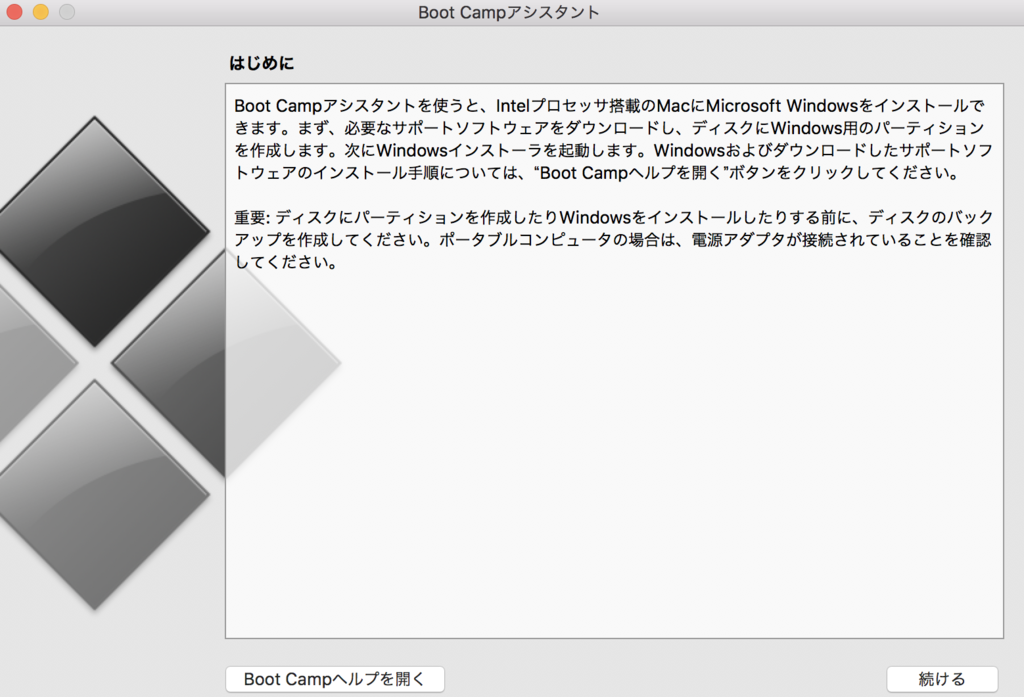この前残業帰りの夜に、ふとホームディレクトリの名前を変えようとした時に,間違ってアカウントの管理者権限を落としてしまった!!

それにbootcampによなおさら拗らせてしまった時の話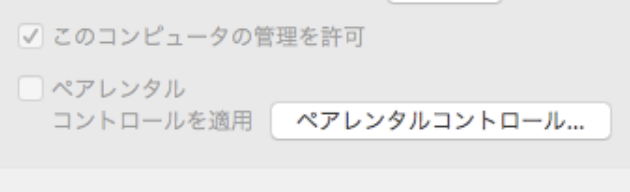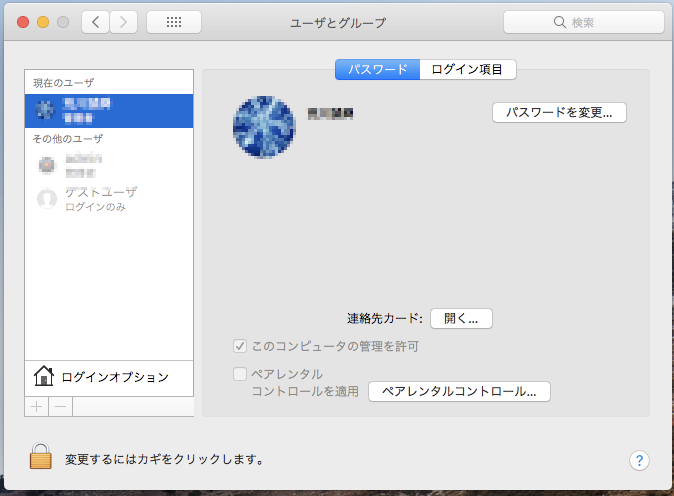ホームディレクトリの名前がクッソダサかったので、変えようとした↓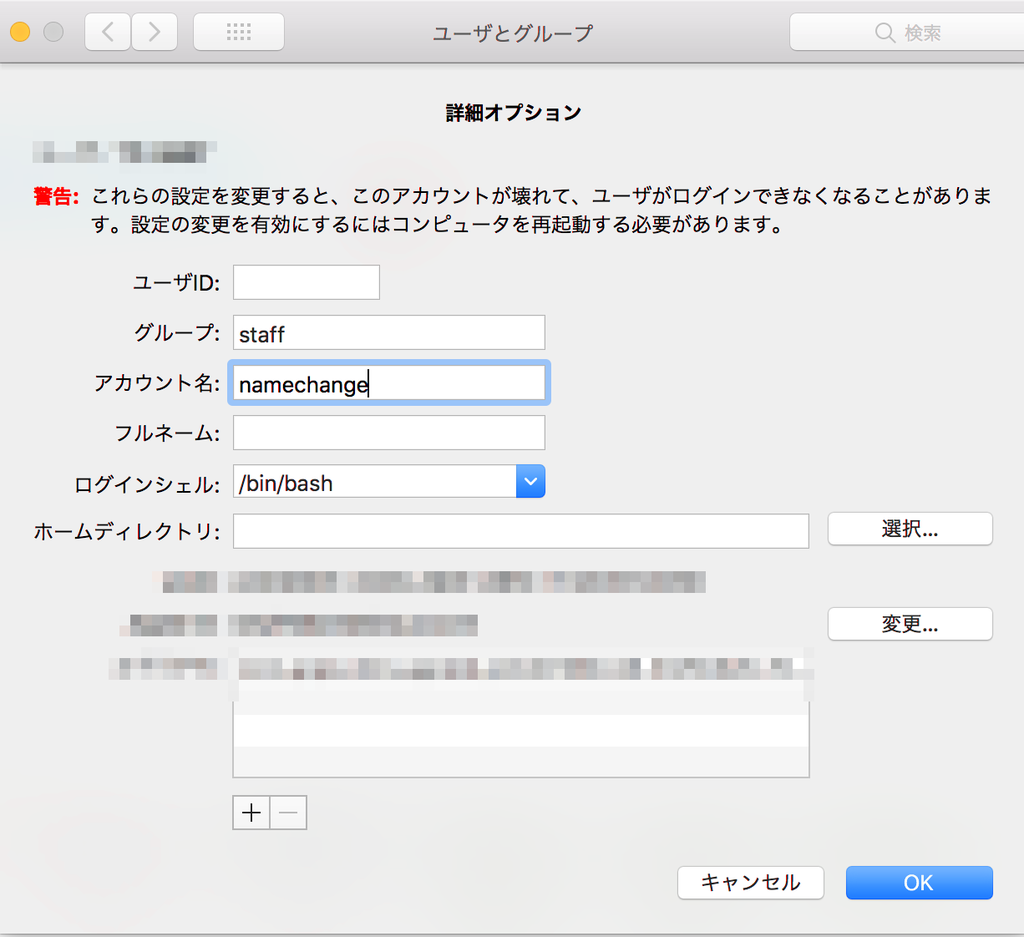ここの管理を許可のチェックが外れていた泣

もちろんパスワードはあってるのに管理者権限がないから開けない

そこでappleさんを見てみた↓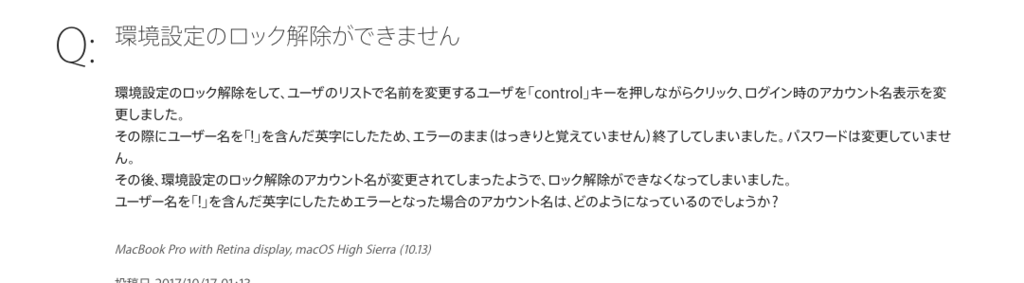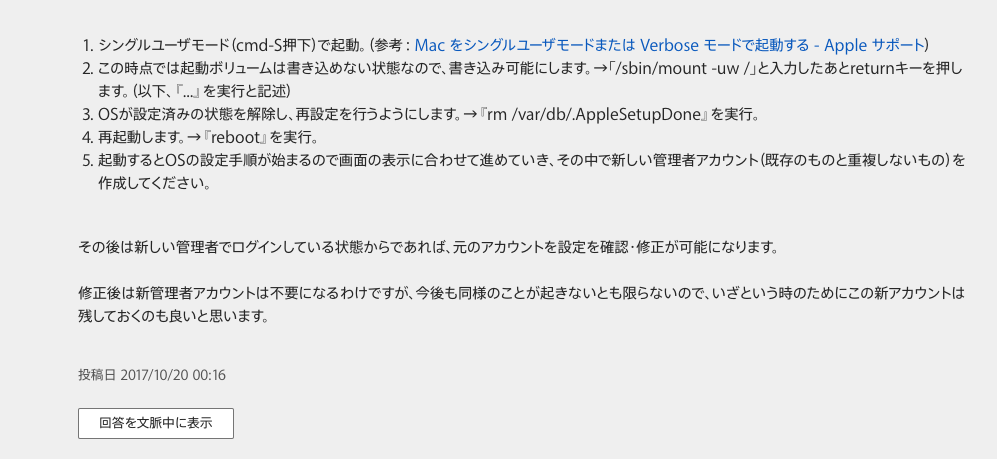うん、なるほど

アップルさんに従ってやってみた。

だが、ここでbootcampさん登場😭

そこで、設定を変更しようとするも管理者権限が必要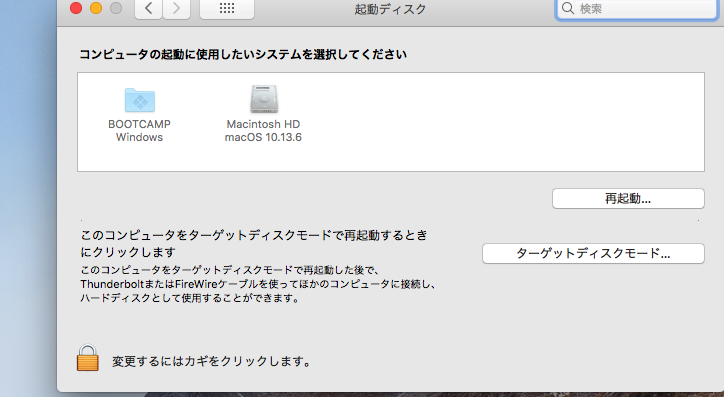じゃあどうする?

＊解決方法↓↓↓

①そのままwindows 10起動しよう

②起動→PCの起動をカスタマイズする→オプションの選択→デバイスの使用→MAC OS

Apple で指示された方法で起動→黒い画面にコマンドを打ち込み新しいアカウント作成完了‼︎これで僕は直りました

もしブートキャンプ入れてる方で管理者権限無くした方はよかったら参考にしてください！

# Laravelで{{\$variable['name'] or なし}}　の変数処理に文字列をたす

どうもお久しぶりです。社畜です

まあ、Laravel始めたばかりなので、ほぼPHPの問題なんのですが

{{\$date['someday'] or なし}}

\$date['someday'] に 9が入っていたなら、9時

なければなしと表示したいわけです。

その場合こうすればできました。

@isset(\$date['someday'])

{{\$date['domeday']}}時

@else

なし

@endisset

これを一行にまとめるとこう!!

↓↓↓

{{isset(\$date['someday']) ? :'なし'}}

とすることで出力できました。

{{\$date['someday'].'時' or　なし }}

{{ \$date['someday'] <?= '時' ?> or なし}}

などをvalidateの問題などが含まれていたりで、試しましたが無理でした。

また、{{}}はhtmlspecialcharsやechoやissetの関数を含んでいたりするので、一見便利ですが使い方が難しかったです。

# 後2日でfuckinサイバー学生生活が再開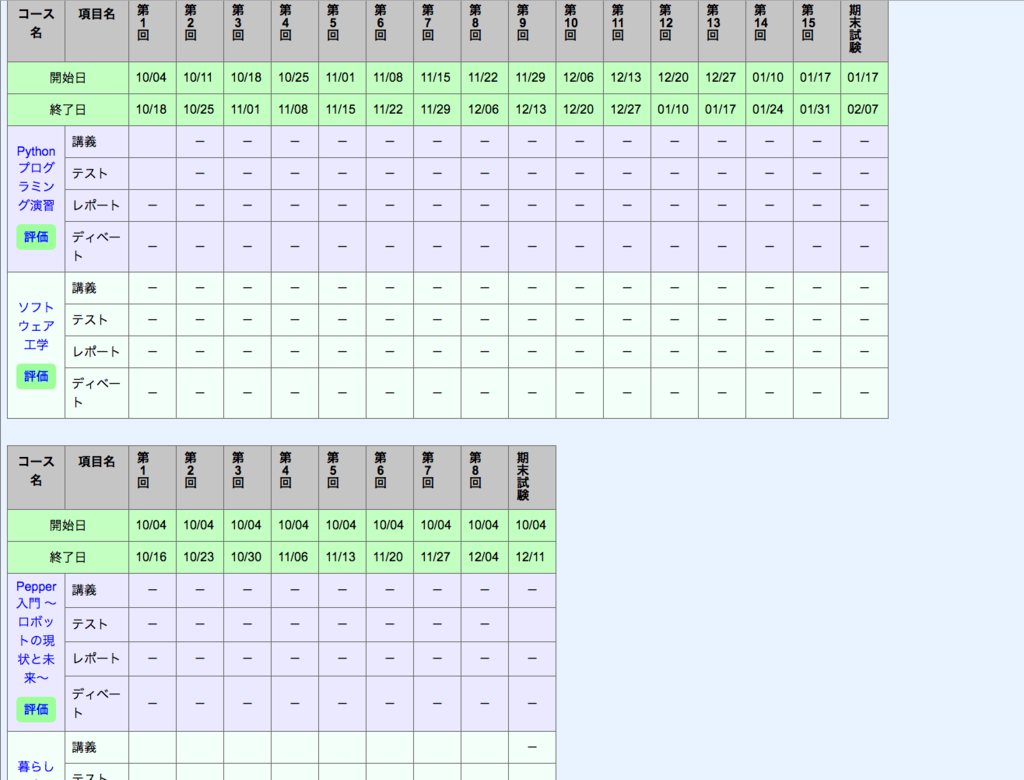• Laravelフレームワークで何か作り始める
• 中国語のビジネスIT用語を覚える
• 基本情報処理資格勉強→まあ、受からんが　時間少なすぎじゃボケ
• Gitの運用を学ぶ

そうせな将来大学院きつすぎるぜ